International
Tables for
Crystallography
Volume B
Reciprocal space
Edited by U. Shmueli

International Tables for Crystallography (2006). Vol. B. ch. 2.5, pp. 278-279   | 1 | 2 |

## Section 2.5.2.2. The interactions of electrons with matter

J. M. Cowleya

#### 2.5.2.2. The interactions of electrons with matter

| top | pdf |

• (1) The elastic scattering of electrons results from the interaction of the charged electrons with the electrostatic potential distribution,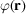, of the atoms or crystals. An incident electron of kinetic energy eW gains energy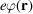in the potential field. Alternatively it may be stated that an incident electron wave of wavelength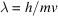is diffracted by a region of variable refractive index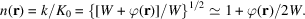• (2) The most important inelastic scattering processes are:

• (a) thermal diffuse scattering, with energy losses of the order of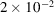eV, separable from the elastic scattering only with specially devised equipment; the angular distribution of thermal diffuse scattering shows variations with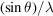which are much the same as for the X-ray case in the kinematical limit;

• (b) bulk plasmon excitation, or the excitation of collective energy states of the conduction electrons, giving energy losses of 3 to 30 eV and an angular range of scattering of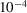to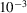rad;

• (c) surface plasmons, or the excitation of collective energy states of the conduction electrons at discontinuities of the structure, with energy losses less than those for bulk plasmons and a similar angular range of scattering;

• (d) interband or intraband excitation of valence-shell electrons giving energy losses in the range of 1 to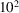eV and an angular range of scattering ofto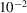rad;

• (e) inner-shell excitations, with energy losses ofeV or more and an angular range of scattering oftorad, depending on the energy losses involved.

• (3) In the original treatment by Bethe (1928)of the elastic scattering of electrons by crystals, the Schrödinger equation is written for electrons in the periodic potential of the crystal; i.e.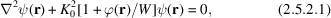where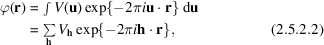K 0 is the wavevector in zero potential (outside the crystal) (magnitude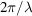) and W is the accelerating voltage. The solutions of the equation are Bloch waves of the form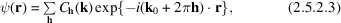where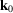is the incident wavevector in the crystal and h is a reciprocal-lattice vector. Substitution of (2.5.2.2)and (2.5.2.3)in (2.5.2.1)gives the dispersion equations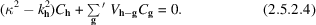Here κ is the magnitude of the wavevector in a medium of constant potential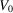(the `inner potential' of the crystal). The refractive index of the electron in the average crystal potential is then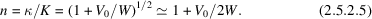Sinceis positive and of the order of 10 V and W is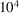to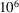V,is positive and of the order of.

Solution of equation (2.5.2.4)gives the Fourier coefficients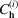of the Bloch waves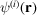and application of the boundary conditions gives the amplitudes of individual Bloch waves (see Chapter 5.2).

• (4) The experimentally important case of transmission of high-energy electrons through thin specimens is treated on the assumption of a plane wave incident in a direction almost perpendicular to an infinitely extended plane-parallel lamellar crystal, making use of the small-angle scattering approximation in which the forward-scattered wave is represented in the paraboloidal approximation to the sphere. The incident-beam direction, assumed to be almost parallel to the z axis, is unique and the z component of k is factored out to give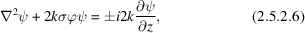where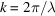and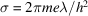. [See Lynch & Moodie (1972), Portier & Gratias (1981), Tournarie (1962), and Chapter 5.2.]

This equation is analogous to the time-dependent Schrödinger equation with z replacing t. Retention of the ± signs on the right-hand side is consistent with both ψ and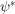being solutions, corresponding to propagation in opposite directions with respect to the z axis. The double-valued solution is of importance in consideration of reciprocity relationships which provide the basis for the description of some dynamical diffraction symmetries. (See Section 2.5.3.)

• (5) The integral form of the wave equation, commonly used for scattering problems, is written, for electron scattering, as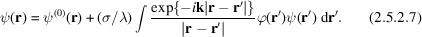The wavefunction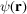within the integral is approximated by using successive terms of a Born series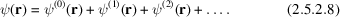The first Born approximation is obtained by putting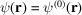in the integral and subsequent terms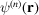are generated by putting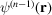in the integral.

For an incident plane wave,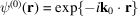and for a point of observation at a large distance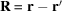from the scattering object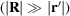, the first Born approximation is generated as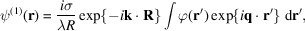where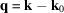or, putting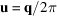and collecting the pre-integral terms into a parameter μ,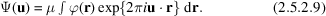This is the Fourier-transform expression which is the basis for the kinematical scattering approximation . It is derived on the basis that allterms for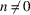are very much smaller than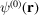and so is a weak scattering approximation.

In this approximation, the scattered amplitude for an atom is related to the atomic structure amplitude,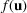, by the relationship, derived from (2.5.2.8),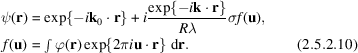For centrosymmetrical atom potential distributions, theare real, positive and monotonically decreasing with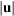. A measure of the extent of the validity of the first Born approximation is given by the fact that the effect of adding the higher-order terms of the Born series may be represented by replacingin (2.5.2.10)by the complex quantities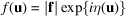and for single heavy atoms the phase factor η may vary from 0.2 for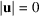to 4 or 5 for large, as seen from the tables of IT C (2004, Section 4.3.3).

• (6) Relativistic effects produce appreciable variations of the parameters used above for the range of electron energies considered. The relativistic values are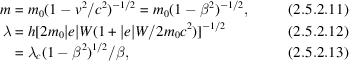where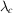is the Compton wavelength,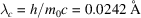, and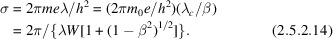Values for these quantities are listed in IT C (2004, Section 4.3.2). The variations of λ and σ with accelerating voltage are illustrated in Fig. 2.5.2.1. For high voltages, σ tends to a constant value,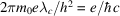.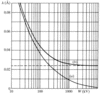Figure 2.5.2.1 | top | pdf |The variation with accelerating voltage of electrons of (a) the wavelength, λ and (b) the quantity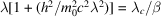which is proportional to the interaction constant σ [equation (2.5.2.14)]. The limit is the Compton wavelength(after Fujiwara, 1961).

### ReferencesInternational Tables for Crystallography (2004). Vol. C. Mathematical, physical and chemical tables, edited E. Prince, 3rd ed. Dordrecht: Kluwer Academic Publishers.Google ScholarBethe, H. A. (1928). Theorie der Beugung von Elektronen an Kristallen. Ann. Phys. (Leipzig), 87, 55–129.Google ScholarLynch, D. F. & Moodie, A. F. (1972). Numerical evaluation of low energy electron diffraction intensity. I. The perfect crystal with no upper layer lines and no absorption. Surf. Sci. 32, 422–438.Google ScholarPortier, R. & Gratias, D. (1981). Diffraction symmetries for elastic scattering. In Electron microscopy and analysis. Inst. Phys. Conf. Ser. No. 61, pp. 275–278. Bristol, London: Institute of Physics.Google ScholarTournaire, M. (1962). Recent developments of the matrical and semi-reciprocal formulation in the field of dynamical theory. J. Phys. Soc. Jpn, 17, Suppl. B11, 98–100.Google Scholar